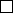3Linear maps

IA Vectors and Matrices3.3 Rank and nullity
Definition
(Rank of linear map)
.
The rank of a linear map
f
:
U V
, denoted
by r(f), is the dimension of the image of f.
Definition
(Nullity of linear map)
.
The nullity of
f
, denoted
n
(
f
) is the
dimension of the kernel of f.
Example.
For the projection onto a plane in
R
3
, the image is the whole plane
and the rank is 2. The kernel is a line so the nullity is 1.
Theorem (Rank-nullity theorem). For a linear map f : U V ,
r(f) + n(f) = dim(U).
Proof.
(Non-examinable) Write
dim
(
U
) =
n
and
n
(
f
) =
m
. If
m
=
n
, then
f
is
the zero map, and the proof is trivial, since
r
(
f
) = 0. Otherwise, assume
m < n
.
Suppose
{e
1
, e
2
, ··· , e
m
}
is a basis of
ker f
, Extend this to a basis of the
whole of
U
to get
{e
1
, e
2
, ··· , e
m
, e
m+1
, ··· , e
n
}
. To prove the theorem, we
need to prove that {f(e
m+1
), f(e
m+2
), ···f(e
n
)} is a basis of im(f ).
(i)
First show that it spans
im
(
f
). Take
y im
(
f
). Thus
x U
such that
y = f(x). Then
y = f(α
1
e
1
+ α
2
e
2
+ ··· + α
n
e
n
),
since e
1
, ···e
n
is a basis of U. Thus
y = α
1
f(e
1
) + α
2
f(e
2
) + ···+ α
m
f(e
m
) + α
m+1
f(e
m+1
) + ···+ α
n
f(e
n
).
The first
m
terms map to
0
, since
e
1
, ···e
m
is the basis of the kernel of
f
.
Thus
y = α
m+1
f(e
m+1
) + ··· + α
n
f(e
n
).
(ii) To show that they are linearly independent, suppose
α
m+1
f(e
m+1
) + ··· + α
n
f(e
n
) = 0.
Then
f(α
m+1
e
m+1
+ ··· + α
n
e
n
) = 0.
Thus
α
m+1
e
m+1
+
···
+
α
n
e
n
ker
(
f
). Since
{e
1
, ··· , e
m
}
span
ker
(
f
),
there exist some α
1
, α
2
, ···α
m
such that
α
m+1
e
m+1
+ ··· + α
n
e
n
= α
1
e
1
+ ··· + α
m
e
m
.
But
e
1
···e
n
is a basis of
U
and are linearly independent. So
α
i
= 0 for all
i
.
Then the only solution to the equation
α
m+1
f
(
e
m+1
) +
···
+
α
n
f
(
e
n
) =
0
is α
i
= 0, and they are linearly independent by definition.
Example.
Calculate the kernel and image of
f
:
R
3
R
3
, defined by
f(x, y, z) = (x + y + z, 2x y + 5z, x + 2z).
First find the kernel: we’ve got the system of equations:
x + y + z = 0
2x y + 5z = 0
x + 2z = 0
Note that the first and second equation add to give 3
x
+6
z
= 0, which is identical
to the third. Then using the first and third equation, we have
y
=
x z
=
z
.
So the kernel is any vector in the form (2z, z, z) and is the span of (2, 1, 1).
To find the image, extend the basis of
ker
(
f
) to a basis of the whole of
R
3
:
{
(
2
,
1
,
1)
,
(0
,
1
,
0)
,
(0
,
0
,
1)
}
. Apply
f
to this basis to obtain (0
,
0
,
0)
,
(1
,
1
,
0)
and (1
,
5
,
2). From the proof of the rank-nullity theorem, we know that
f
(0
,
1
,
0)
and f (0, 0, 1) is a basis of the image.
To get the standard form of the image, we know that the normal to the plane
is parallel to (1
,
1
,
0)
×
(1
,
5
,
2)
k
(1
,
1
,
3). Since
0 im
(
f
), the equation of
the plane is x + y 3z = 0.